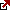# Present Value Annuity Factor

The present value annuity factor is the ratio used to calculate the present value of the future one dollar cash flows. The rule is that the higher the discount rate is, the lower the present value of the annuity.

The present value plays an important role in the annuity factor. “R” represents rate per period while “N” represents number per period. The rate per period is equal in importance to the number per period. Both of these variables are very important for accurately solving the equation. The overall present value annuity factor is used to solve and calculate future cash flows. These future cash flows are very important to solve as they help to find the solution to the present value. This formula relies heavily on the concept of time, specifically the time value of funds. The time value of funds continuous to play a large role in the success of an equation.

Present Value Annuity Factor = 1-( 1+r)^-n / r where r is the rate per period and n number of periods.

The time value of funds is also known as the time value of money. Time value is a specific idea relating to the value of funds at a determined time. This type of concept is one of the best ways to determine the practical value of funds. Values are subject to change over time as a result of external factors. Therefore it is important to calculate the time value of funds/money. An amount of money today can be reserved and invested for future earnings. Periodic cash flow is also another important concept used in order to help determine the solution. The original amount of the loan also plays a role in the solution to the equation.

The main goal of the present value annuity formula is to fully simplify the process of calculating. A table is often used to help find the solution to the present value annuity factor equation. The present value annuity factor is one of the best methods for calculating accurate information and stats for the overall outcome of the equation in relation to time value. There are many examples of this type of equation. One example of this equation would be \$500 annual payments for a total of five years. \$500 annual payments can be made for a total of five years. This means that once a year, \$500 dollars would be due in order to successfully uphold the financial agreement. This is very important to note as the \$500 annual payments are the best way to continuously meet the time value of funds invested or agreed upon.

There are two main ways to look at this type of equation. The first way to look at this equation is to look for the present value annuity. The 5% increase over 5 years will also play a role in the overall outcome of this equation. The end result will allow \$500 to be multiplied by 4.32 in order to arrive at the final solution to the equation. \$500 multiplied by 4.32 will produce an answer of \$2,164. This is the final answer to the equation which will allow for the investment or financial agreement to be completed using the present value annuity factor successfully. Therefore this type of equation has many uses and practical applications on a day to day basis in the financial industry as well as other types of investments.

Latest posts by Fxigor (see all)

Fxigor

Igor has been a trader since 2007. Currently, Igor works for several prop trading companies. He is an expert in financial niche, long-term trading, and weekly technical levels. The primary field of Igor's research is the application of machine learning in algorithmic trading. Education: Computer Engineering and Ph.D. in machine learning. Igor regularly publishes trading-related videos on the Fxigor Youtube channel. To contact Igor write on: igor@forex.in.rs

Trade gold and silver. Visit the broker's page and start trading high liquidity spot metals - the most traded instruments in the world.Diversify your savings with a gold IRA.

Gold & silver portfolio-building assistance from product professionals.

If you want to trade stocks try: